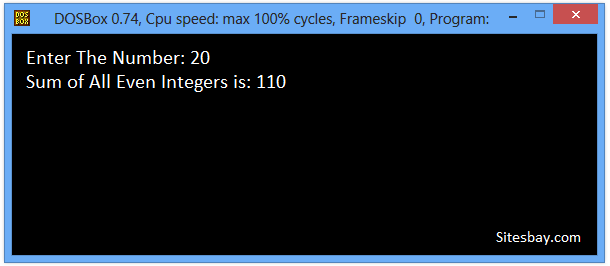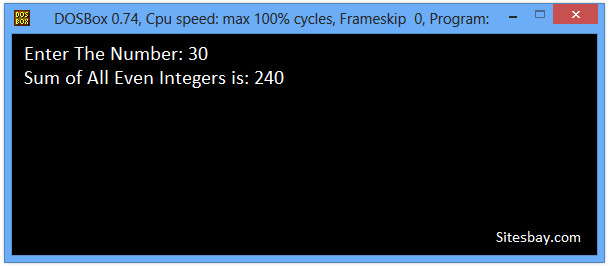# Find Sum of Even Integers Proram in C

## C Program to Calculate Sum of Even Integers

To write this code we need to know How to check number is ever or odd in C. First user enter upper limit to process this code.

### Logic to Find Sum of Even Numbers

• Input upper limit to find sum of all even numbers and store it in some variable say N.
• Initialize another variable to store sum with 0 say sum = 0.
• To find sum of even numbers we need to iterate through even numbers from 1 to n. Initialize a loop from 2 to N and increment 2 on each iteration. The loop structure should look like for(i=2; i<=N; i+=2).
• Inside the loop body add previous value of sum with i i.e. sum = sum + i.
• After loop print final value of sum.

Note: Operator sum += i is equivalent to sum = sum + i.

## Find Sum of All Even Integers Program in C

```#include<stdio.h>
#include<conio.h>

void main()
{
int i, n, sum=0;
clrscr();

scanf("%d", &n);

for(i=2; i<=n; i+=2)
{
//Adding current even number to sum variable

sum = sum + i
}

printf("Sum of All Even Number Between 1 to %d = %d", n, sum);
getch();
}
```

## Output

```Enter The Number: 20
Sum of All Even Integers is: 110
```## Find Sum of All Even Integers Program in C

```#include<stdio.h>
#include<conio.h>

void main()
{
int i,n,sum;
sum=0;
clrscr();
printf("Enter The Number: ");
scanf("%d",&n);
for(i=1; i<=n; i++)
{
if(i%2==0)
sum=sum+i;
}
printf("Sum of All Even Integers is: %d",sum);
getch();
}
```

## Output

```Enter The Number: 30
Sum of All Even Integers is: 240
```WIFI CCTV Camera